# Loan Calculator in Microsoft Excel

In this article, we will learn how to make EMI calculator or Loan calculator in Microsoft Excel.

EMI stands for Equated Monthly Installment. This is fixed amount paid by the borrower to financier and this amount is used to pay for both interest and principal each month.

In today’s world, nearly every person has had a Loan from a bank in several forms. When we want to get an overview or a plan of how a loan would be paid back then this template is the best in which we need to fill details like Loan Amount, Rate of Interest per annum and Number of Installments. After filling this information automatically, we will get detailed description of how a loan would be paid back and what amount would get disbursed in principal amount and interest amount every month. Also, it would give the EMI amount.

The image below shows the template of the EMI of a bank.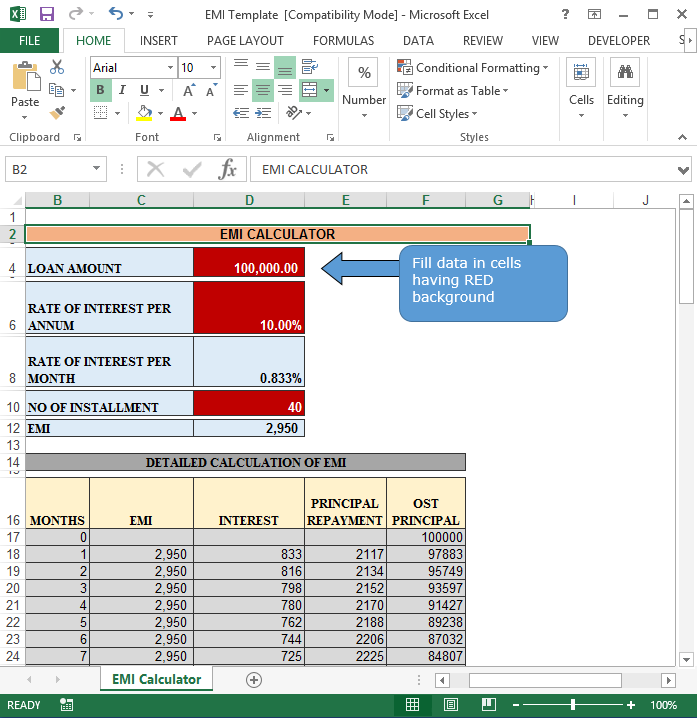The image below shows where we need to fill the data:-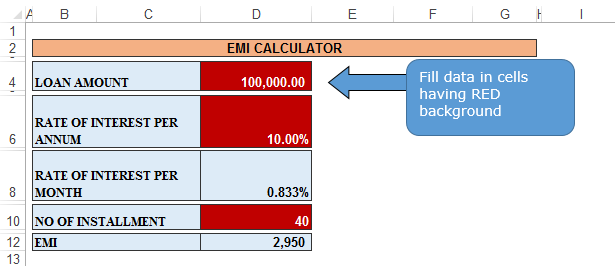In the red cells, we need to insert the loan amount, rate of interest per month and number of installments. This template would calculate rate of interest per month and EMI

In cell D4, we need to write the loan amount taken from the bank as shown in below screenshot.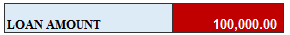In cell D6, we need to write the rate of interest we need to pay on account of loan amount withdrawn from a bank as shown in below screenshot.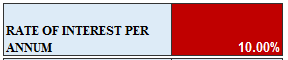In cell D8, automatically the rate of interest per month would be calculated as shown in below screenshot.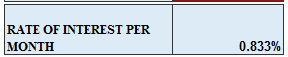In cell D10, we need to write the number of installments we need to pay to the bank as shown in below screenshot.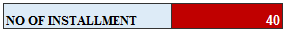In cell D12 automatically the EMI’s which are to be paid per month would be calculated as shown in below screenshot.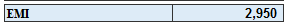The formula we use to calculate the EMI amount =-PMT(D8,D10,D4)

The image below shows the automated calculations. These columns would calculate the months, EMI, Interest to be paid, principal repayment and OST principal.

Calculation Points:-

1. EMI = D12
2. Interest = Rate of Interest per month* OST Principal
3. Principal Repayment = EMI – Interest
4. OST Principal = Last Month’s OST Principal – Current Month’s Principal Repayment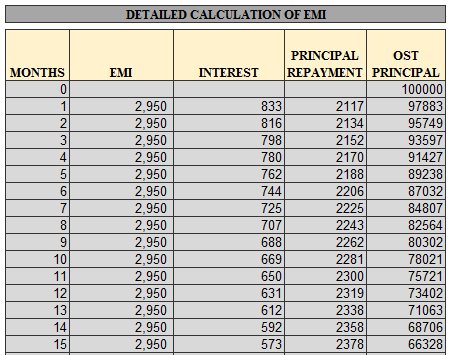This template gives us a clear idea of how the loan dues has to be cleared, in which month the dues would be paid and what amount of EMI will be paid in interest and principal amount.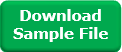1.